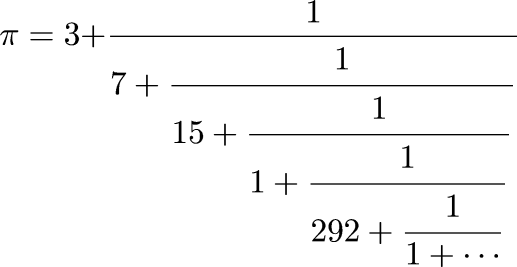Next Continued Fraction Arithmetic 16

# Continued Fractions• The typography is a bit overwhelming

• We write: π = [3; 7, 15, 1, 292, 1, ...]

• In general, we have a = [a0; a1, a2, ...]

• a0 is the integer part of a; α is the fractional part a - a0

• a1, a2, ... is the continued fraction expansion of 1/(a-a0)

• So [a0; a1, a2, ...] = a0 + 1/[a1; a2, ...]

• a0 can be any integer

• But for i ≥ 1, ai is necessarily positive

• With this restriction, the continued fraction representation is unique (almost)

 Next Back